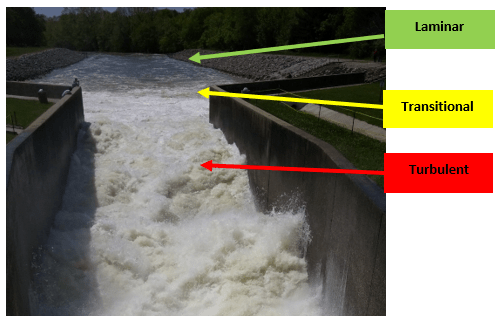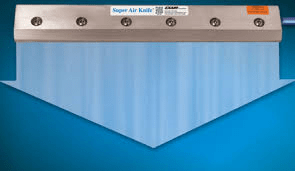## Boundary Layer: Laminar and Turbulent flow

Fluid mechanics is the field that studies the properties of fluids in various states.  Fluid dynamics studies the forces on a fluid, either as a liquid or a gas, during motion.  Osborne Reynolds, an Irish innovator, popularized this dynamic with a dimensionless number, Re. This number determines the state in which the fluid is moving; either laminar flow, transitional flow, or turbulent flow.  For compressed air, Re < 2300 will have laminar flow while Re > 4000 will have turbulent flow.  Equation 1 below shows the relationship between the inertial forces of the fluid as compared to the viscous forces.

Equation 1:

Re = V * Dh / u

Re – Reynolds Number (no dimensions)

V – Velocity (feet/sec or meters/sec)

Dh – hydraulic diameter (feet or meters)

u – Kinematic Viscosity (feet^2/sec or meter^2/sec)

To dive deeper into this, we will need to examine the boundary layer.  The boundary layer is the area that is near the surface of the object.  This could refer to a wing on an airplane or a blade from a turbine.  In this blog, I will target pipes, tubes, and hoses that are used for transporting fluids.  The profile across the area (reference diagram below) is a velocity gradient.  The boundary layer is the distance from the wall or surface to 99% of the maximum velocity of the fluid stream.  At the surface, the velocity of the fluid is zero because the fluid is in a “no slip” condition.  As we move away from the wall, the velocity starts to increase.  The boundary layer distance measures that area where the velocity is not uniform.  If you reach 99% of the maximum velocity very close to the wall of the pipe, the air flow is turbulent.  If the boundary layer reaches the radius of the pipe, then the velocity is fully developed, or laminar.Boundary Layer Concept

The calculation is shown in Equation 2.

Equation 2:

d = 5 * X / (Re1/2)

d – Boundary layer thickness (feet or meter)

X – distance in pipe or on surface (feet or meter)

Re – Reynolds Number (no dimensions) at distance X

This equation can be very beneficial for determining the thickness where the velocity is not uniform along the cross-section.  As an analogy, imagine an expressway as the velocity profile, and the on-ramp as the boundary layer.  If the on-ramp is long and smooth, a car can reach the speed of traffic and merge without disrupting the flow.  This would be considered Laminar Flow.  If the on-ramp is curved but short, the car has to merge into traffic at a much slower speed.  This will disrupt the flow of some of the traffic.  I would consider this as the transitional range.  Now imagine an on-ramp to be very short and perpendicular to the expressway. As the car goes to merge into traffic, it will cause chaos and accidents.  This is what I would consider to be turbulent flow.EXAIR Digital Flowmeter

In a compressed air system, similar things happen within the piping scheme.  Valves, tees, elbows, pipe reducers, filters, etc. are common items that will affect the flow.  Let’s look at a scenario with the EXAIR Digital Flowmeters.  In the instruction manual, we require the meter to be placed 30 pipe diameters from any disruptions.  The reason is to get a laminar air flow for accurate flow measurements.  In order to get laminar flow, we need the boundary layer thickness to reach the radius of the pipe.  So, let’s see how that number was calculated.

Within the piping system, high Reynold’s numbers generate high pressure drops which makes the system inefficient.  For this reason, we should keep Re < 90,000.  As an example, let’s look at the 2” EXAIR Digital Flowmeter.  The maximum flow range is 400 SCFM (standard cubic feet per min).  In looking at Equation 2, the 2” Digital Flowmeter is mounted to a 2” Sch40 pipe with an inner diameter of 2.067” (52.5mm).  The radius of this pipe is 1.0335” (26.2 mm) or 0.086 ft (0.026m).  If we make the Boundary Layer Thickness equal to the radius of the pipe, then we will have laminar flow.  To solve for X which is the distance in the pipe, we can rearrange the terms to:

X = d * (Re)1/2 / 5 = 0.086ft * (90,000)1/2 / 5 = 5.16 ft or 62”

If we look at this number, we will need 62” of pipe to get a laminar air flow for the worse-case condition.  If you know the Re value, then you can change that length of pipe to match it and still get valid flow readings.  From the note above, the Digital Flowmeter will need to be mounted 30 pipe diameters.  So, the pipe diameter is 2.067” and at 30 pipe diameters, we will need to be at 30 * 2.067 = 62”.  So, with any type of common disruptions in the air stream, you will always get good flow data at that distance.

Why is this important to know?  In many compressed air applications, the laminar region is the best method to generate a strong force efficiently and quietly.  Allowing the compressed air to have a more uniform boundary layer will optimize your compressed air system.  And for the Digital Flowmeter, it helps to measure the flow correctly and consistently.  If you would like to discuss further how to reduce “traffic jams” in your process, an EXAIR Application Engineer will be happy to help you.

John Ball
Application Engineer
Email: johnball@exair.com
Twitter: @EXAIR_jb

## Video Blog: Laminar and Turbulent Flows

I have written blogs about laminar and turbulent flows as related to the Reynold’s number.  Now, let’s demonstrate the difference between the two flows and the advantages of laminar flow from EXAIR’s engineered air nozzles; as demonstrated by our VariBlast Safety Air Gun.

John Ball
Application Engineer
Email: johnball@exair.com
Twitter: @EXAIR_jb

## Laminar Flow Compared to Turbulent Flowturbulent vs laminar

Fluid mechanics is the field that studies the properties of fluids in various states.  There are two main areas; fluid statics and fluid dynamics.  Fluid dynamics studies the forces on a fluid, either as a liquid or a gas, during motion.  Osborne Reynolds, an Irish innovator, popularized this dynamic with a dimensionless number, Re. This number determines the state in which the fluid is moving; either laminar flow, transitional flow, or turbulent flow.  Equation 1 below shows the relationship between the inertial forces of the fluid as compared to the viscous forces.

Equation 1:  Re = V * Dh/u

Re – Reynolds Number (no dimensions)

V – Velocity (feet/sec or meters/sec)

Dh – hydraulic diameter (feet or meters)

u – Kinematic Viscosity (feet^2/sec or meter^2/sec)

The value of Re will mark the region in which the fluid (liquid or gas) is moving.  If the Reynolds number, Re, is below 2300, then it is considered to be laminar (streamline and predictable).  If Re is greater than 4000, then it is considered to be turbulent (chaotic and violent).  The area between these two numbers is the transitional area where you can have eddy currents and some non-linear velocities.  To better show the differences between each state, I have a picture below that shows water flowing from a drain pipe into a channel.  The water is loud and disorderly; traveling in different directions, even upstream.  With the high velocity of water coming out of the drain pipe, the inertial forces are greater than the viscous forces of the water.  This indicates turbulent flow with a Reynolds number larger than 4000.  As the water flows into the mouth of the river, the waves transform from a disorderly mess into a more uniform stream.  This is the transitional region.  A bit further downstream, the stream becomes calm and quiet, flowing in the same direction.  This is laminar flow.  Air is also a fluid, and it will behave in a similar way depending on the Reynolds number.Turbulent to Laminar Water

Why is this important to know?  In certain applications, one state may be better suited than the other.  For mixing, suspension and heat transfer; turbulent flows are better.  But, when it comes to effective blowing, lower pressure drops and reduced noise levels; laminar flows are better.  In many compressed air applications, the laminar region is the best method to generate a strong force efficiently and quietly.  EXAIR offers a large line of products, including the Super Air Knives, Super Air Amplifiers and Super Air Nozzles that utilizes that laminar flow for compressed air applications.  If you would like to discuss further how laminar flows could benefit your process, an EXAIR Application Engineer will be happy to help you.

John Ball
Application Engineer
Email: johnball@exair.com

Twitter: @EXAIR_jb

## What is Laminar Flow and Turbulent Flow?Super Air Knife

Fluid mechanics is the field that studies the properties of fluids in various states.  There are two areas, fluid statics and fluid dynamics.  Fluid dynamics studies the forces on a fluid, either as a liquid or a gas, during motion.  Osborne Reynolds, an Irish innovator, popularized this dynamic with a dimensionless number, Re. This number determines the state in which the fluid is moving; either laminar flow or turbulent flow.  Equation 1 shows the relationship between the inertial forces of the fluid as compared to the viscous forces.

Equation 1:  Re = V * Dh/u

Re – Reynolds Number (no dimensions)

V – Velocity (feet/sec or meters/sec)

Dh – hydraulic diameter (feet or meters)

u – Kinematic Viscosity (feet^2/sec or meter^2/sec)

The value of Re will mark the region in which the fluid (liquid or gas) is moving.  If the Reynolds number, Re, is below 2300, then it is considered to be laminar (streamline and predictable).  If Re is greater than 4000, then it is considered to be turbulent (chaotic and violent).  The area between these two numbers is the transitional area where you can have eddy currents and some non-linear velocities.  To better show the differences between each state, I have a picture below that shows water flowing from a drain pipe into a channel.  The water is loud and disorderly; traveling in different directions, even upstream.  With the high velocity of water coming out of the drain pipe, the inertial forces are greater than the viscosity of the water.  This indicates turbulent flow with a Reynolds number larger than 4000.  As the water flows into the mouth of the river after the channel, the waves transform from a disorderly mess into a more uniform stream.  This is the transitional region.  A bit further downstream, the stream becomes calm and quiet, flowing in the same direction.  This is laminar flow.  Air is also a fluid, and it will behave in a similar way depending on the Reynolds number.Turbulent to Laminar Water

Why is this important to know?  In certain applications, one state may be better suited than the other.  For mixing, suspension and heat transfer; turbulent flows are better.  But, when it comes to effective blowing, lower pressure drops and reduced noise levels; laminar flows are better.  In many compressed air applications, the laminar region is the best method to generate a strong force efficiently and quietly.  EXAIR offers a large line of products, including the Super Air Knives and Super Air Nozzles that utilizes that laminar flow for compressed air applications.  If you would like to discuss further how laminar flows could benefit your process, an EXAIR Application Engineer will be happy to help you.

John Ball
Application Engineer
Email: johnball@exair.com
Twitter: @EXAIR_jb